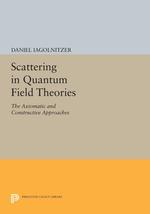# Scattering in Quantum Field Theories: The Axiomatic and Constructive Approaches

Daniel Iagolnitzer
Pages: 312
https://www.jstor.org/stable/j.ctt7ztp4s

1. Front Matter
(pp. i-iv)
(pp. v-viii)
3. Preface
(pp. ix-xii)
4. Introduction
(pp. xiii-2)

The aim of this introduction is to present an overall view of the organization and contents of the book, which will complement indications given in the Preface. Contents will be described in Sect. 2 and some brief technical remarks are given in Sect. 3. More details and explanations on the various topics treated, as also an historical survey and references, will be found in the introductory section of each chapter.

The book is composed of four chapters. Chapters II, III and IV are concerned with the first, second and third themes mentioned in the Preface, namely, scattering theory in axiomatic...

5. CHAPTER I The Multiparticle S Matrix
(pp. 3-54)

The aim of this chapter is to introduce the scattering operatorS,orSmatrix, independently of field theory and to present general properties that can be expected on various rigorous or heuristic grounds, with emphasis on various aspects of interest developed in the 1970s and 1980s, which will not be found in standard books of field theory. As mentioned in the Preface, we restrict our attention to systems of massive particles with short-range interactions. Our purpose is mainly descriptive. Links between various properties will be mentioned but we do not try to start from a minimal number of principles...

6. CHAPTER II Scattering Theory in Axiomatic Field Theory
(pp. 55-148)

Let us first recall the traditional Wightman axioms in Minkowski space-time. This framework will be somewhat modified and completed for our purposes, but it can be considered as the fundamental starting point. Wightman axioms apply to field operatorsA(x)acting on a Hilbert spaceHof physical states (to be later interpreted asymptotically in terms of particles in theories of interest);$x = ({x_0},\vec x)$is a real space-time variable and eachAis more precisely an operator-valued distribution which, to each test functionf, associates an operatorA(f)(= “∫ A(x)f(x)d(x)”)inH. (The fieldsAof interest here might be defined in...

7. CHAPTER III Euclidean Constructive Field Theory
(pp. 149-207)

As mentioned in Sect. 3 of the general Introduction, notations are different throughout this part from those of Chapter II:xorpwill be taken real in Euclidean space, with${x^2} = x_0^2 + {\vec x^2},{p^2} = p_0^2 + {\vec p^2}.$

The purpose of this chapter is to present the Euclidean definition of models, and related results, in nonperturbative field theory. We start in Sects. 1.2 and 1.3 with some preliminaries. Sect. 1.2 presents the standard Euclidean axioms [OS,GJ] that are believed to be satisfied by any probability measure dμ(φ)(on the space of distributionsφin Euclidean space-time) characterizing a field theory in the class we wish to...

8. CHAPTER IV Particle Analysis in Constructive Field Theory
(pp. 208-270)

We start in Sect. 1.1 with some indications on the perturbative approach and will then give a more precise introduction to the constructive (and semi-axiomatic) analysis in Sect. 1.2. With regard to notations used in this chapter, see Sect. 3.2 of the general Introduction.

As explained in Sect. 2.1 of Chapter III, (connected)N-point functions appear in the perturbative approach as formal infinite sums of possibly renormalized Feynman integrals, with possibly renormalized coupling constants at each vertex. (For simplicity, we leave aside the effective expansions mentioned in Sect. 2.2 of Chapter III.) We consider these series, initially, in Euclidean energy-momentum...

9. Mathematical Appendix: Distributions, analytic functions, and microlocal analysis
(pp. 271-278)
10. Bibliography
(pp. 279-280)
11. References
(pp. 281-286)
12. Index
(pp. 287-290)